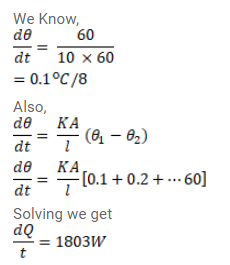# A rod of negligible heat capacity has length

Question:

A rod of negligible heat capacity has length $20 \mathrm{~cm}$, area of cross - section $1.0 \mathrm{~cm}^{2}$ and thermal conductivity $200 \mathrm{~W} / \mathrm{m}-{ }^{\circ} \mathrm{C}$ The temperature of one end is maintained at $0^{\circ} \mathrm{C}$ and that of the other end is slowly and linearly varied from $0^{\circ} \mathrm{C}$ to $60^{\circ} \mathrm{C}$ in 10 minutes. Assuming no loss of heat through the sides, find the total heat transmitted through the rod in these 10 minutes.

Solution: